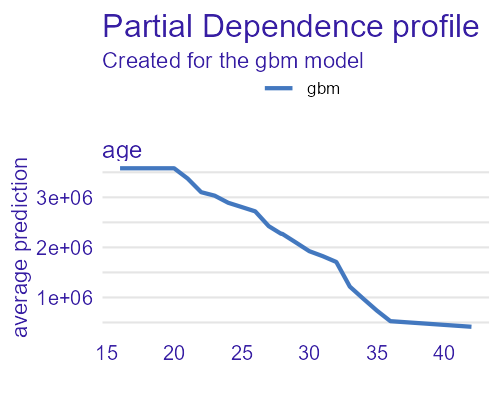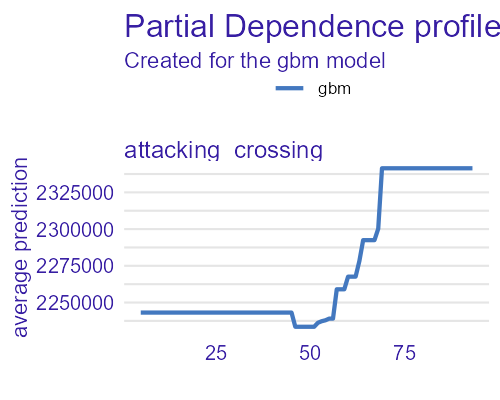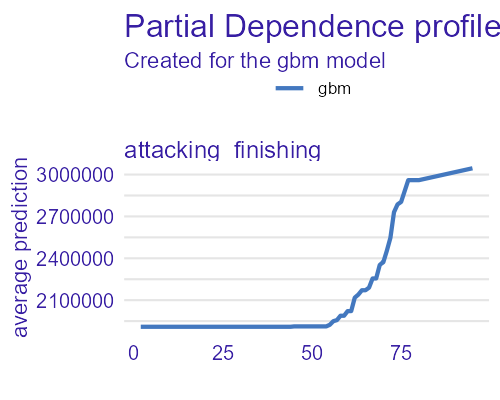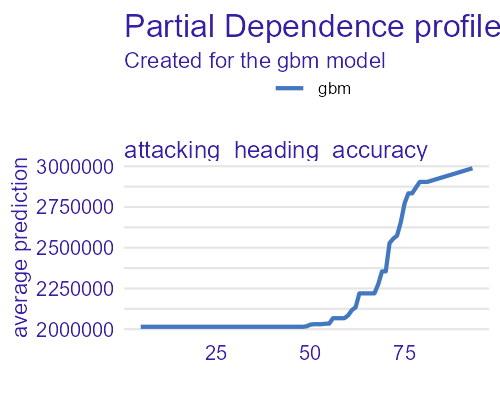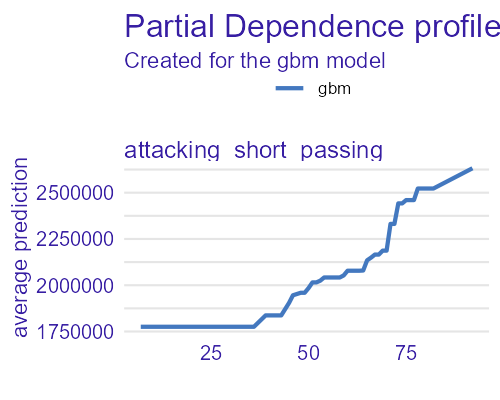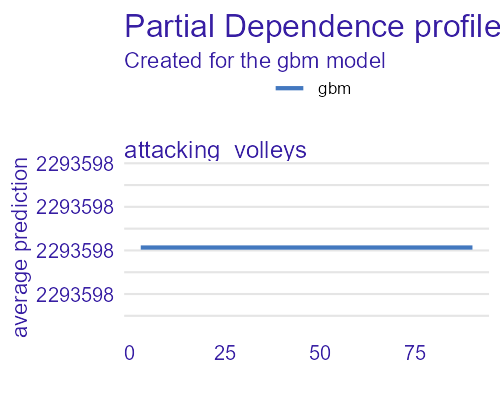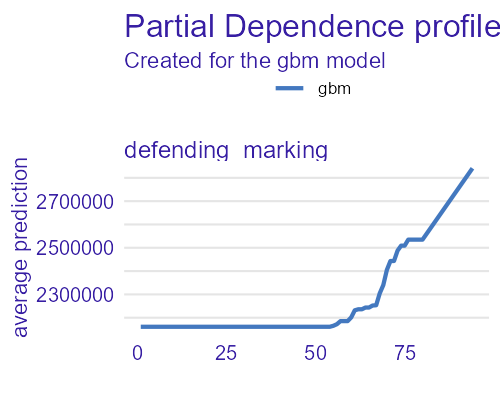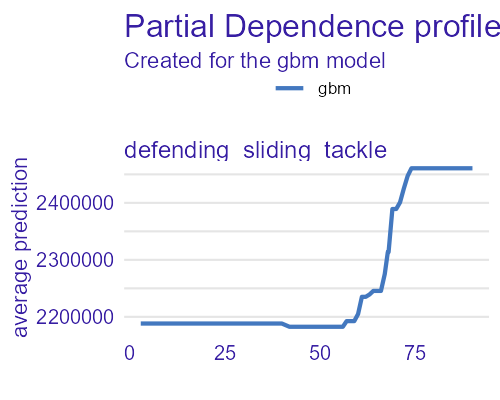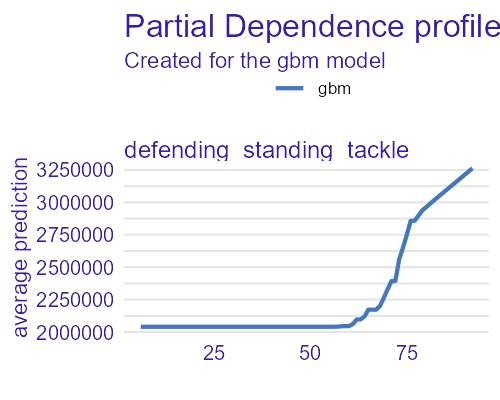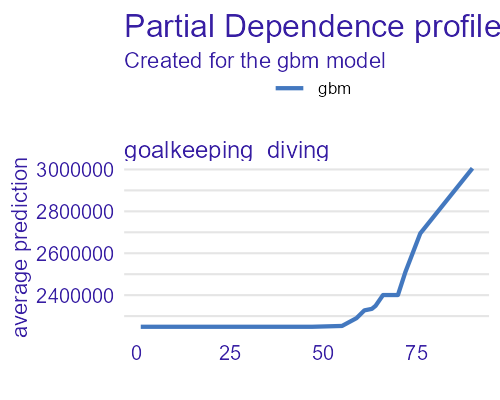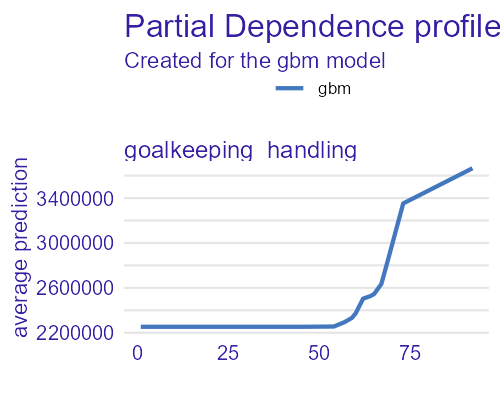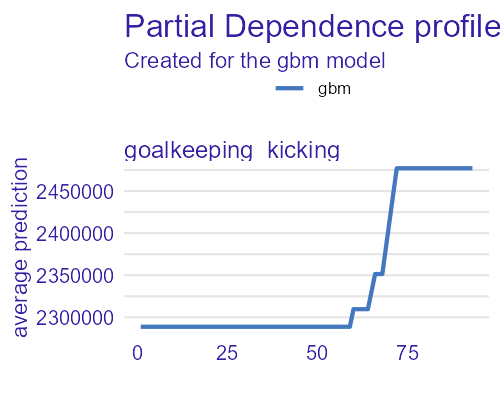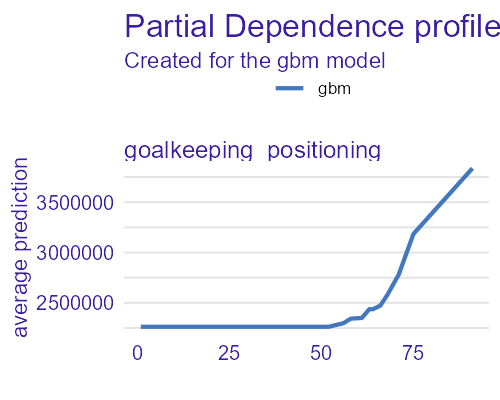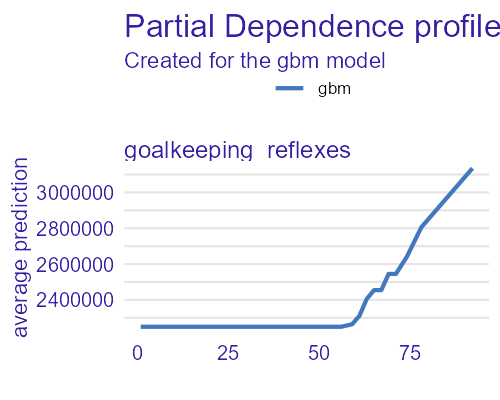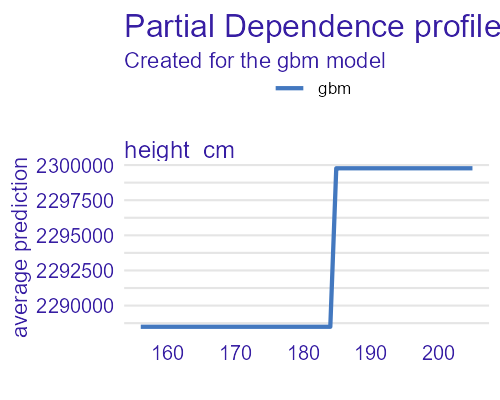## The problem

Data analysis (and creating models) involves many stages. For early exploration, it is useful to have a grip not only on individual series (AKA variables) available, but also on relations between them. Unfortunately, the task of understanding correlations between variables proves to be difficult ($$n$$ variables means $$n(n-1) / 2$$ pairs of variables). Furthermore, the mainstream method of visualizing them (i.e. correlation matrix) has its limits; the more variables, the less readable (and therefore meaningful) it becomes.

## Package corrgrapher

This package aims to plot correlations between variables in form of a graph. Variables correlated with each other shall be close (positively and negatively alike), and weakly correlated - far from each other.

It is achieved through a physical simulation, where the nodes are treated as points with mass (and are pushing each other away) and edges are treated as mass-less springs. The length of a spring depends on absolute value of correlation between connected nodes. The bigger the correlation, the shorter the spring.

## Example 1 - Seatbelts

Let’s take a look at one of datasets available in R - Seatbelts. It contains information about road casualties in Great Britain in 1969-84 period, and has following columns:

• DriversKilled - amount of car drivers killed
• drivers - amount of car drivers killed or seriously injured
• front - amount of front-seat passengers killed or seriously injured
• rear - amount of rear-seat passengers killed or seriously injured
• kms - distance driven
• PetrolPrice - petrol price
• VanKilled - number of van drivers killed.
• law - binary variable; was the law enforcing seatbelt use in effect?

For our purposes, since Pearson’s correlation index is irrelevant for binary variables, we drop the law variable.

Thanks to implementation of knit_print() method, an object of class corrgrapher can be displayed simply by calling it:

library('corrgrapher')
df <- as.data.frame(datasets::Seatbelts)[,-8] # Drop the binary variable
cgr <- corrgrapher(df)

Thanks to implementation of knit_print() method, an object of class corrgrapher can be displayed simply by calling it:

cgr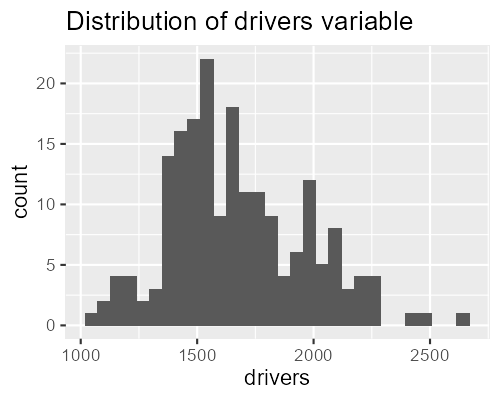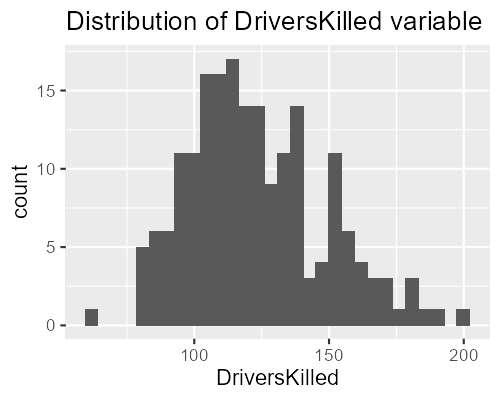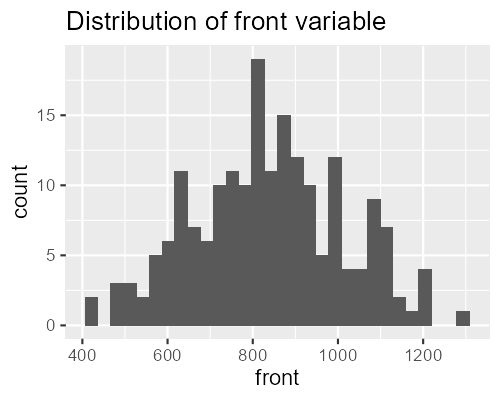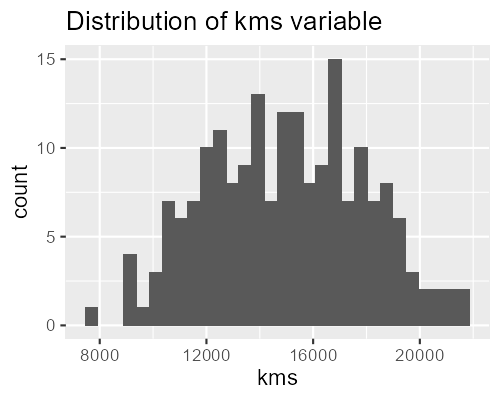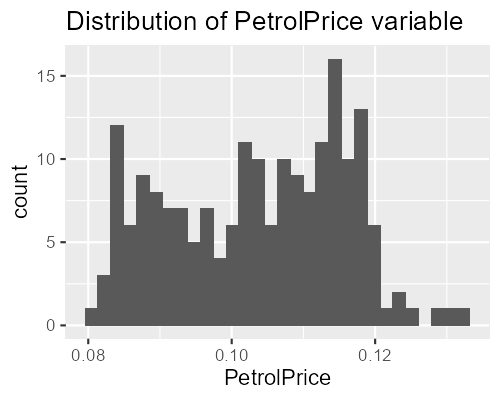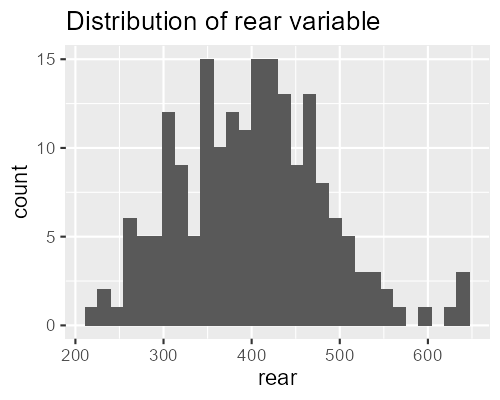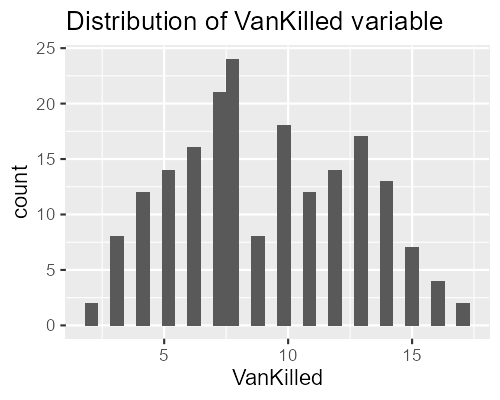On the side a simple plot with distribution of variables is displayed. The figure is interactive - feel free to select a variable from a drag-drop selector or to click on the node.

As expected, we see, that all variables regarding casualties are correlated with each other, but rear and VanKilled weaker than others. We also observe the negative correlation between PetrolPrice and variables drivers, DriversKilled and front.

## Comparable correlation coefficients

Calculating comparable correlation coefficients between variables of different types (numerical - numerical, numerical - categorical, categorical - categorical) is a non-trivial problem. In this package, when encountering data with different kinds of variables, a following methodology is used:

1. First, the $$p$$-values are calculated of 3 different statistical tests:
• Pearson’s correlation test (cor.test) for 2 numerical variables
• Kruskal’s test (kruskal.test) for a numerical and categorical variable
• Chi-squared test (chisq.test) for 2 categorical variables
1. Then, the $$-log_{10}(p)$$ is calculated and treated as ma measure of correlation between variables. All results above 100 ($$p$$-value < $$10^{-100}$$) are treated as absolute correlation and their value is reduced to 100

2. Finally, results are scaled to fit inside $$[0,1]$$ .

## Example 2 - Titanic

Dataset with information about passengers of Titanic is a good example of dataset with both numerical (age, fare, sibsp, parch) and categorical(gender, embarked, country, survived) data. Let us build a model to predict, whether a passenger survived the sinking or not.

Here, let us introduce to a way of combining the CorrGrapheR package with packages from DrWhyAI family. The CorrrapheR function may take an explainer object (created with the help of DALEX package), extract the data from it, and add extra features to the displayed figure.

The visualization is enriched with:

• Incorporation of importance of variables for the output of the model, using the size of nodes. The bigger the nodes, the more important they are
• Partial dependency plots, displayed on the side.
library(ranger)
library(ingredients)
library(DALEX)
data("titanic_imputed", package='DALEX')
tit_model <- ranger(survived ~ ., data = titanic_imputed, num.trees = 100)
tit_model_exp <- explain(tit_model,
data = titanic_imputed[,-8],
y = titanic_imputed[, 8],
verbose = FALSE)
tit_cgr <- corrgrapher(tit_model_exp)
tit_cgr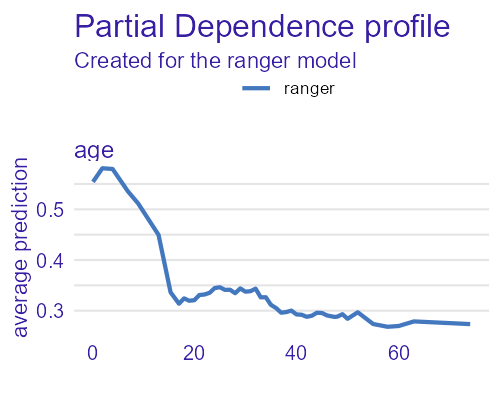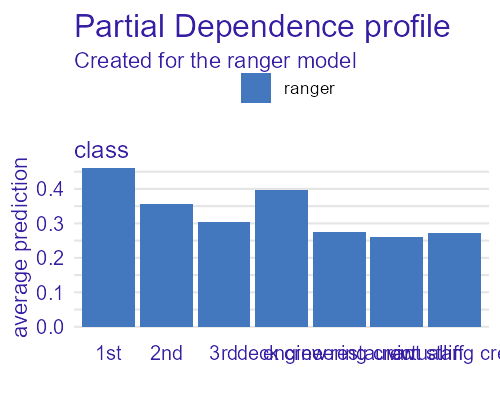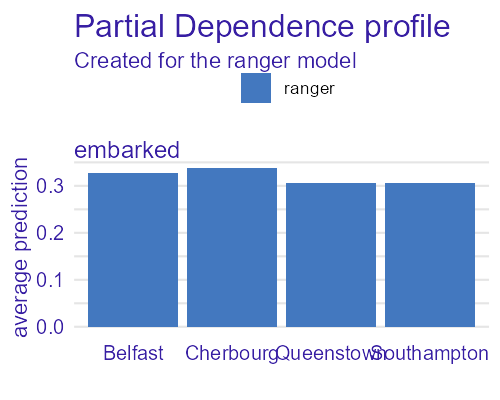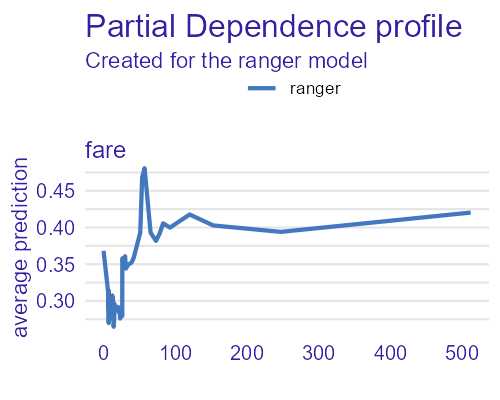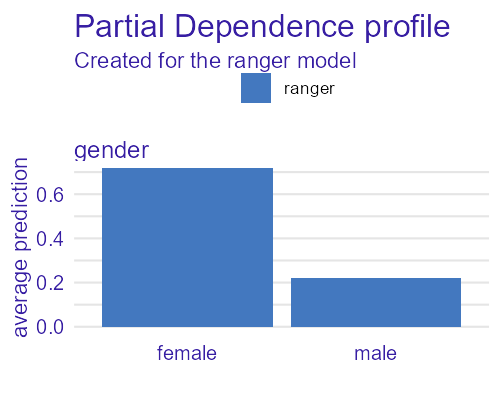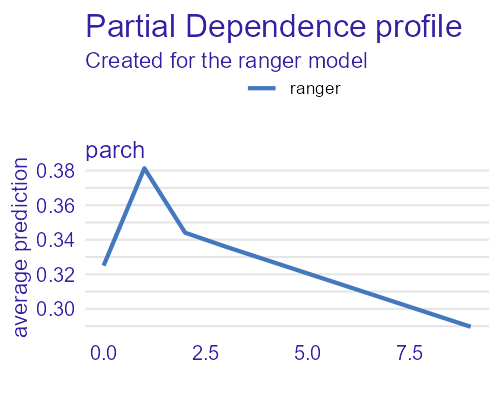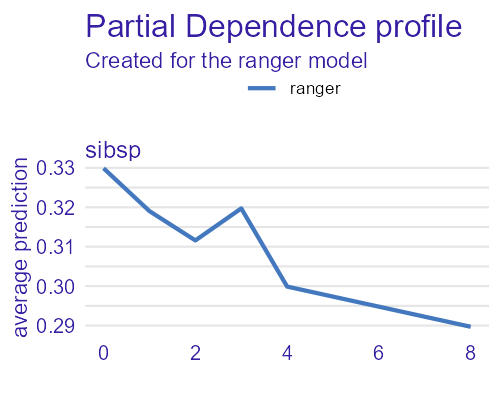What can we learn from the figure:

• The most important variable is gender - women were given priority to access evacuation boats.
• Right after it are variables class (1st class was privileged) and age (children also were given priority during evacuation).
• We also see, that fare was an important variable, but it was very strongly connected to class variable - no surprise there.
• Surprisingly, class is strongly correlated with gender and embarked.
• Finally, parch (amount of parents/children aboard) and sibsp(amount of spouses/siblings abroad) are strongly connected. It would indicate, that traveling with whole family was a common occurance.

## Example 3 - FIFA

Let’s look at something more challenging to visualize. The dataset for FIFA 20 soccer game (more info here and here) contains 89 columns of data about soccer players from all around the world. Visualizing it is a non-trivial task.

For this use-case, let us create a model based on numerical variables (42 in total), that will predict the value in EUR of soccer players.

library("gbm")

fifa20_selected <- fifa20[,c(4,5,7,8,11:13,17,26,45:78)]

# Value is skewed. Will be much easier to model sqrt(Value).

fifa20_selected$value_eur <- log10(fifa20_selected$value_eur)
fifa20_selected <- na.omit(fifa20_selected)
fifa20_selected <- fifa20_selected[fifa20_selected$value_eur > 0,] fifa20_selected <- fifa20_selected[!duplicated(fifa20_selected[,1]),] rownames(fifa20_selected) <- fifa20_selected[,1] fifa20_selected <- fifa20_selected[,-1] # create a gbm model set.seed(1313) # 4:5 are overall and potential, too strong predictors fifa_gbm <- gbm(value_eur ~ . , data = fifa20_selected[,-(4:5)], n.trees = 250, interaction.depth = 3) # Create DALEX explainer fifa_gbm_exp <- DALEX::explain(fifa_gbm, data = fifa20_selected[, -6], y = 10^fifa20_selected$value_eur,
predict_function = function(m,x)
10^predict(m, x, n.trees = 250))

fifa_feat <- ingredients::feature_importance(fifa_gbm_exp)
fifa_pd <- ingredients::partial_dependency(fifa_gbm_exp)
# Finally, create a corrgrapher object
fifa_cgr <- corrgrapher(fifa_gbm_exp, cutoff = 0.4,
feature_importance = fifa_feat,
partial_dependency = list(numerical = fifa_pd))
fifa_cgr